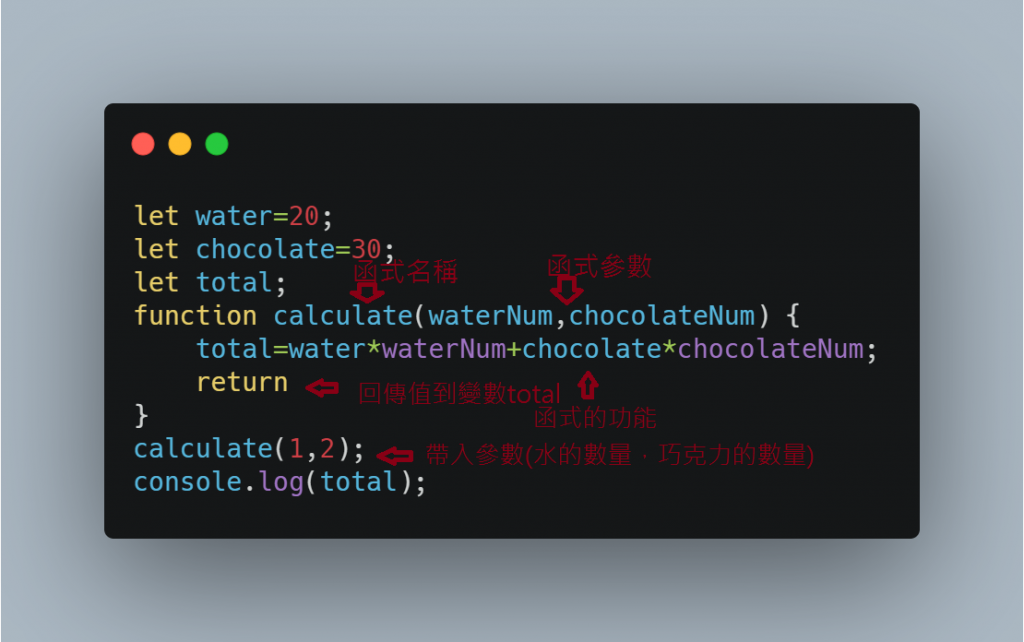#0

## 鼠年全馬鐵人挑戰 WEEK 20: JavaScript 函式 function

### 函式

• 函式名稱
• 函式參數 `()`
• 函式功能 `{}`
``````let water=20;
let chocolate=30;
let total;
function calculate(waterNum,chocolateNum) {
total=water*waterNum+chocolate*chocolateNum;
return
}
calculate(1,2);
console.log(total);
````````````let water=20;
let chocolate=30;
function calculate(waterNum,chocolateNum) {
let total;
total=water*waterNum+chocolate*chocolateNum;
if (total>=50){
return console.log(`恭喜獲得一支古早味冰棒`)
}else{
return console.log(`你的消費不夠，還需要在消費！`)
}
}
calculate(1,2);
``````

`函式 function`就是把重複用到的功能打包成一個`函式 function`